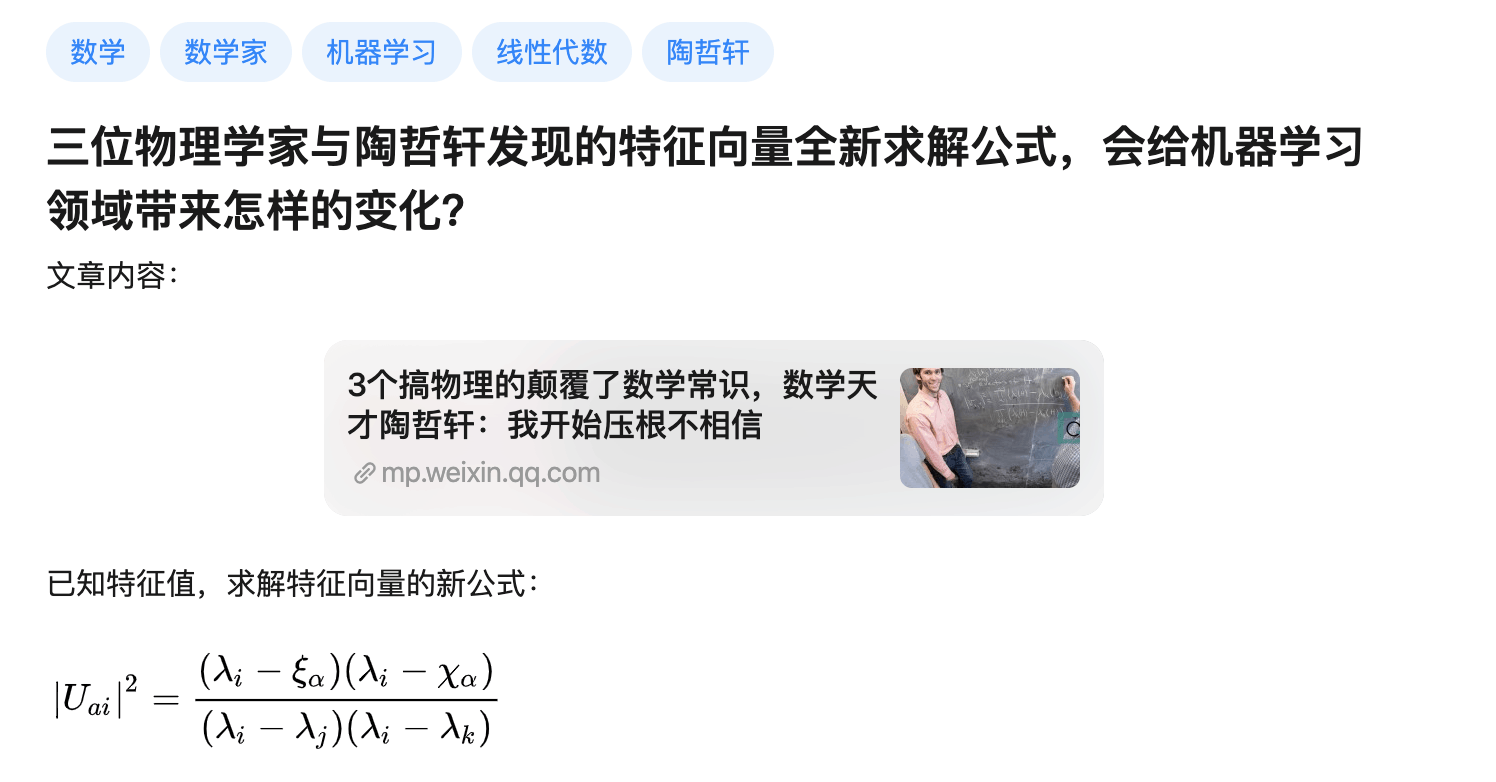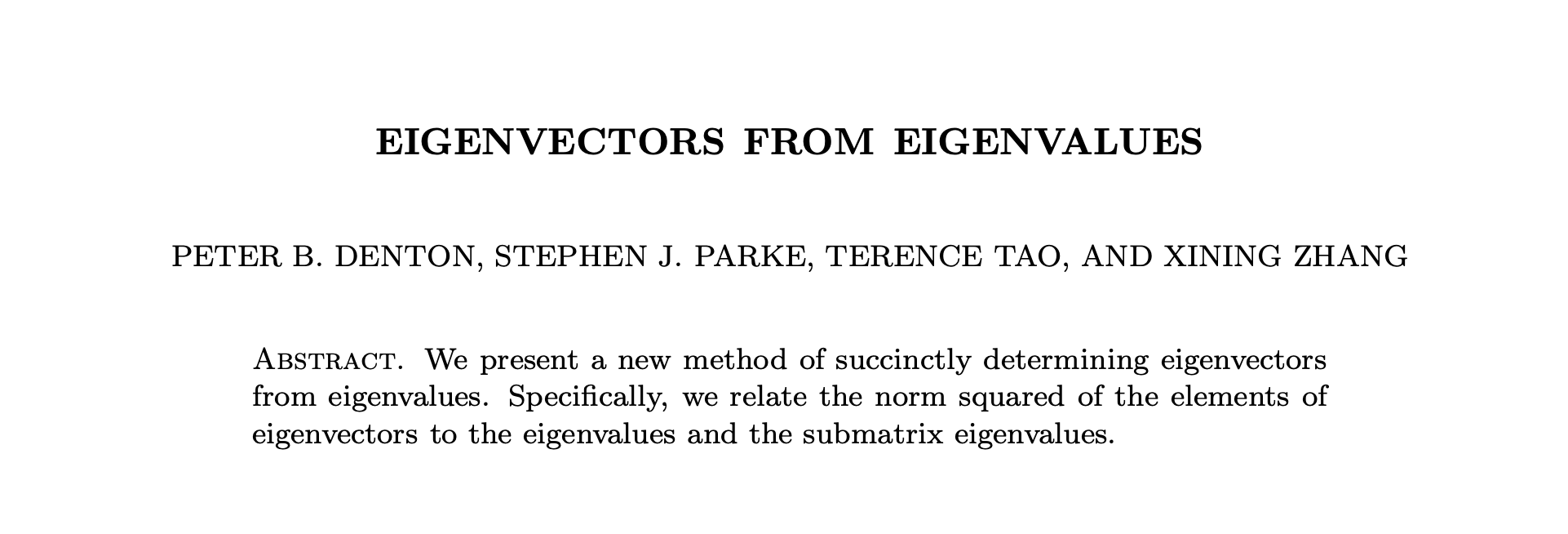2019 年 11 月 30 日

## 不明觉厉## 疑问重重\left|v_{i, j}\right|^{2} =\frac{\prod_{k=1}^{n-1}\left(\lambda_{i}(A)-\lambda_{k}\left(M_{j}\right)\right)}{\prod_{k=1 ; k \neq i}^{n}\left(\lambda_{i}(A)-\lambda_{k}(A)\right)}